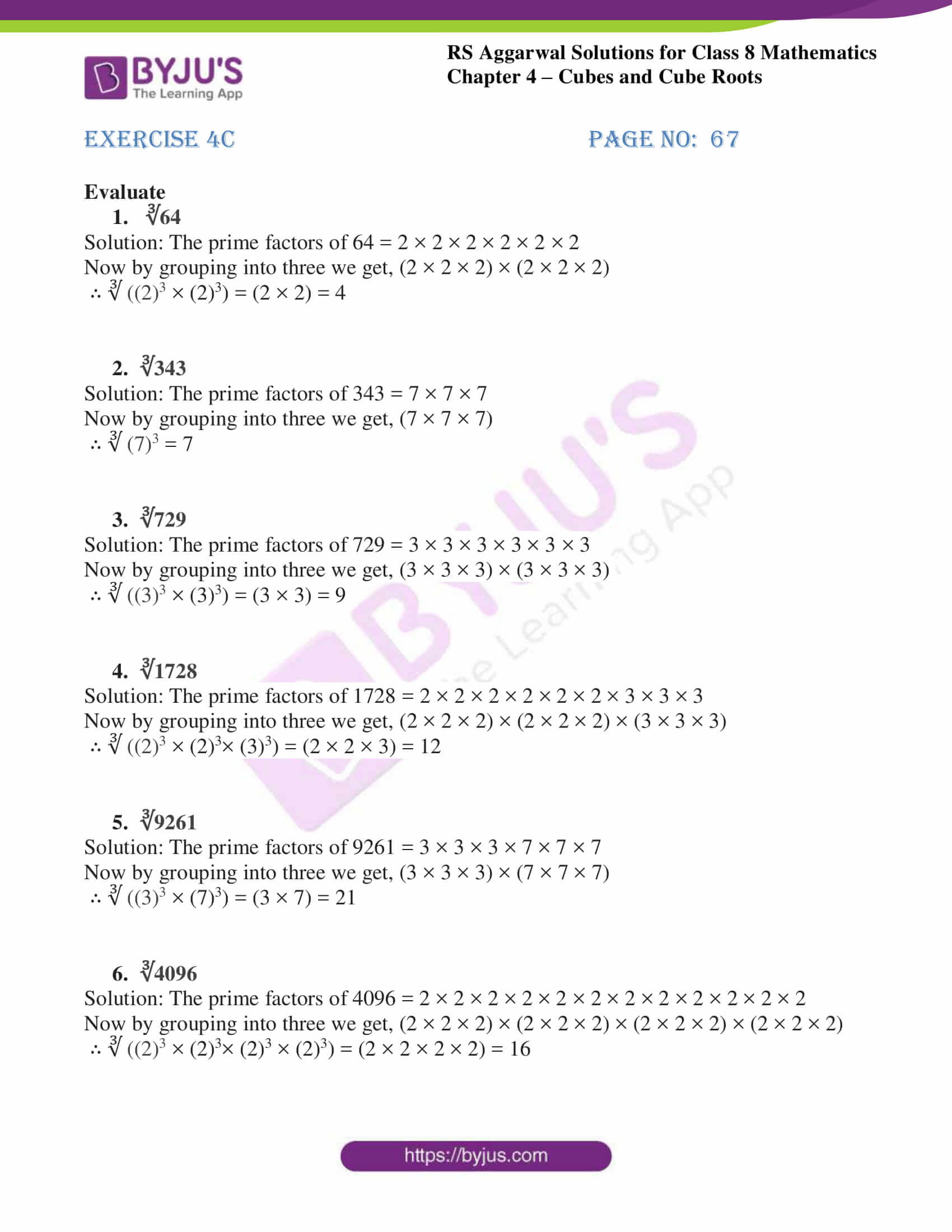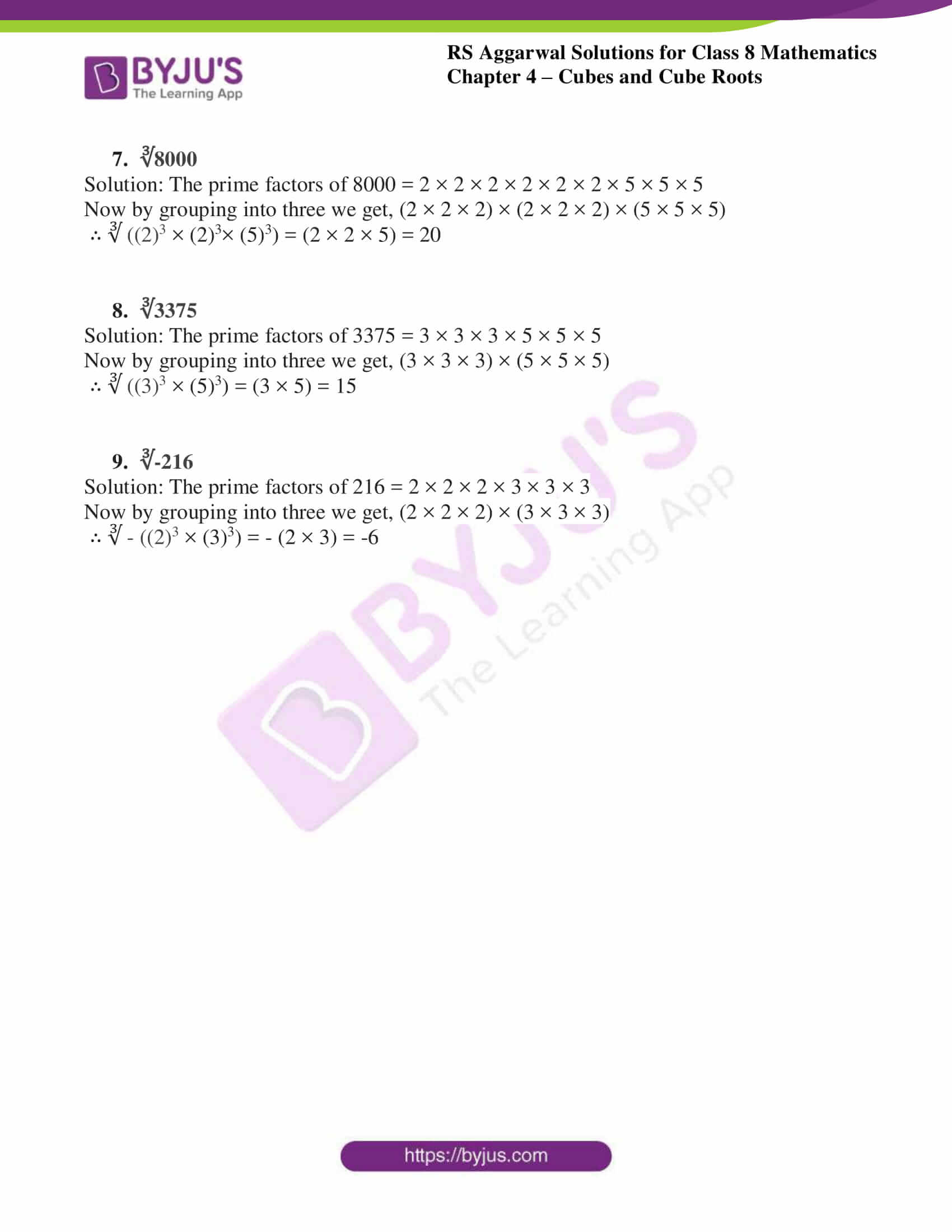# RS Aggarwal Solutions for Class 8 Maths Chapter 4 - Cubes and Cube Roots Exercise 4C

RS Aggarwal Solutions for Class 8 Maths Chapter 4- Exercise 4C, Cubes and Cube Roots can be downloaded by the links provided below. Our experts have solved the RS Aggarwal Solutions to help students in learning the fundamentals and also ensure that the students are thorough with their basic concepts by practicing the solutions.

In Exercise 4C of RS Aggarwal Class 8 Maths, we shall study how the cube roots are solved by using the factorisation method. cube root of a negative perfect cube, cube root of the product of integers and cube root of a rational number.

## Download PDF of RS Aggarwal Solutions for Class 8 Maths Chapter 4- Cubes and Cube Roots Exercise 4C### Access Answers to RS Aggarwal Solutions for Class 8 Maths Chapter 4- Cubes and Cube Roots Exercise 4C

Evaluate

1. ∛64

Solution: The prime factors of 64 = 2 × 2 × 2 × 2 × 2 × 2

Now by grouping into three we get, (2 × 2 × 2) × (2 × 2 × 2)

∴ ∛ ((2)3 × (2)3) = (2 × 2) = 4

2. ∛343

Solution: The prime factors of 343 = 7 × 7 × 7

Now by grouping into three we get, (7 × 7 × 7)

∴ ∛ (7)3 = 7

3. ∛729

Solution: The prime factors of 729 = 3 × 3 × 3 × 3 × 3 × 3

Now by grouping into three we get, (3 × 3 × 3) × (3 × 3 × 3)

∴ ∛ ((3)3 × (3)3) = (3 × 3) = 9

4. ∛1728

Solution: The prime factors of 1728 = 2 × 2 × 2 × 2 × 2 × 2 × 3 × 3 × 3

Now by grouping into three we get, (2 × 2 × 2) × (2 × 2 × 2) × (3 × 3 × 3)

∴ ∛ ((2)3 × (2)3× (3)3) = (2 × 2 × 3) = 12

5. ∛9261

Solution: The prime factors of 9261 = 3 × 3 × 3 × 7 × 7 × 7

Now by grouping into three we get, (3 × 3 × 3) × (7 × 7 × 7)

∴ ∛ ((3)3 × (7)3) = (3 × 7) = 21

6. ∛4096

Solution: The prime factors of 4096 = 2 × 2 × 2 × 2 × 2 × 2 × 2 × 2 × 2 × 2 × 2 × 2

Now by grouping into three we get, (2 × 2 × 2) × (2 × 2 × 2) × (2 × 2 × 2) × (2 × 2 × 2)

∴ ∛ ((2)3 × (2)3× (2)3 × (2)3) = (2 × 2 × 2 × 2) = 16

7. ∛8000

Solution: The prime factors of 8000 = 2 × 2 × 2 × 2 × 2 × 2 × 5 × 5 × 5

Now by grouping into three we get, (2 × 2 × 2) × (2 × 2 × 2) × (5 × 5 × 5)

∴ ∛ ((2)3 × (2)3× (5)3) = (2 × 2 × 5) = 20

8. ∛3375

Solution: The prime factors of 3375 = 3 × 3 × 3 × 5 × 5 × 5

Now by grouping into three we get, (3 × 3 × 3) × (5 × 5 × 5)

∴ ∛ ((3)3 × (5)3) = (3 × 5) = 15

9. ∛-216

Solution: The prime factors of 216 = 2 × 2 × 2 × 3 × 3 × 3

Now by grouping into three we get, (2 × 2 × 2) × (3 × 3 × 3)

∴ ∛ – ((2)3 × (3)3) = – (2 × 3) = -6

### Access other Exercises of RS Aggarwal Solutions for Class 8 Maths Chapter 4- Cubes and Cube Roots

Exercise 4A Solutions 10 Questions

Exercise 4B Solutions 4 Questions

Exercise 4D Solutions 10 Questions

## RS Aggarwal Solutions for Class 8 Maths Chapter 4- Cubes and Cube Roots Exercise 4C

Exercise 4C of RS Aggarwal Class 8, Cubes and Cube Roots contains advance concepts related to Cubes. This exercise mainly deals with cube roots. Cube roots of a given number are solved by using the prime factorisation method where the given number is expressed as the product of primes. Grouping them into triplets and then finding the product of those triplets results in the solution of the cube root.

The RS Aggarwal Solutions can help the students practice at ease while learning the concepts as it provides all the answers to the questions from the RS Aggarwal textbook. Students should practice RS Aggarwal Solutions without fail to understand how the concepts are solved, which in turn helps in increasing their confidence level to achieve high marks.